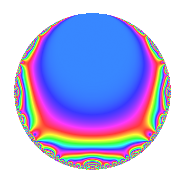Properties

 Label 9450.2.a.ewLevel 9450 Weight 2 Character orbit 9450.a Self dual Yes Analytic conductor 75.459 Analytic rank 1 Dimension 2 CM No Inner twists 1

Related objects

Newspace parameters

 Level: $$N$$ = $$9450 = 2 \cdot 3^{3} \cdot 5^{2} \cdot 7$$ Weight: $$k$$ = $$2$$ Character orbit: $$[\chi]$$ = 9450.a (trivial)

Newform invariants

 Self dual: Yes Analytic conductor: $$75.4586299101$$ Analytic rank: $$1$$ Dimension: $$2$$ Coefficient field: $$\Q(\sqrt{10})$$ Coefficient ring: $$\Z[a_1, \ldots, a_{11}]$$ Coefficient ring index: $$1$$ Fricke sign: $$1$$ Sato-Tate group: $\mathrm{SU}(2)$

$q$-expansion

Coefficients of the $$q$$-expansion are expressed in terms of $$\beta = \sqrt{10}$$. We also show the integral $$q$$-expansion of the trace form.

 $$f(q)$$ $$=$$ $$q$$ $$+ q^{2}$$ $$+ q^{4}$$ $$+ q^{7}$$ $$+ q^{8}$$ $$+O(q^{10})$$ $$q$$ $$+ q^{2}$$ $$+ q^{4}$$ $$+ q^{7}$$ $$+ q^{8}$$ $$+ ( 2 + \beta ) q^{11}$$ $$+ ( -1 - \beta ) q^{13}$$ $$+ q^{14}$$ $$+ q^{16}$$ $$-5 q^{17}$$ $$+ ( -4 - \beta ) q^{19}$$ $$+ ( 2 + \beta ) q^{22}$$ $$+ ( -3 - \beta ) q^{23}$$ $$+ ( -1 - \beta ) q^{26}$$ $$+ q^{28}$$ $$+ ( 1 + \beta ) q^{29}$$ $$+ ( 1 + 3 \beta ) q^{31}$$ $$+ q^{32}$$ $$-5 q^{34}$$ $$+ ( -6 + \beta ) q^{37}$$ $$+ ( -4 - \beta ) q^{38}$$ $$+ ( -4 - 2 \beta ) q^{41}$$ $$-7 q^{43}$$ $$+ ( 2 + \beta ) q^{44}$$ $$+ ( -3 - \beta ) q^{46}$$ $$+ 2 q^{47}$$ $$+ q^{49}$$ $$+ ( -1 - \beta ) q^{52}$$ $$+ ( 3 - 3 \beta ) q^{53}$$ $$+ q^{56}$$ $$+ ( 1 + \beta ) q^{58}$$ $$-7 q^{59}$$ $$+ ( -2 + 2 \beta ) q^{61}$$ $$+ ( 1 + 3 \beta ) q^{62}$$ $$+ q^{64}$$ $$+ ( -9 + 2 \beta ) q^{67}$$ $$-5 q^{68}$$ $$+ ( -3 - 3 \beta ) q^{71}$$ $$-10 q^{73}$$ $$+ ( -6 + \beta ) q^{74}$$ $$+ ( -4 - \beta ) q^{76}$$ $$+ ( 2 + \beta ) q^{77}$$ $$+ ( 10 + \beta ) q^{79}$$ $$+ ( -4 - 2 \beta ) q^{82}$$ $$+ ( 2 - 2 \beta ) q^{83}$$ $$-7 q^{86}$$ $$+ ( 2 + \beta ) q^{88}$$ $$+ ( -9 + 2 \beta ) q^{89}$$ $$+ ( -1 - \beta ) q^{91}$$ $$+ ( -3 - \beta ) q^{92}$$ $$+ 2 q^{94}$$ $$+ ( 2 + \beta ) q^{97}$$ $$+ q^{98}$$ $$+O(q^{100})$$ $$\operatorname{Tr}(f)(q)$$ $$=$$ $$2q$$ $$\mathstrut +\mathstrut 2q^{2}$$ $$\mathstrut +\mathstrut 2q^{4}$$ $$\mathstrut +\mathstrut 2q^{7}$$ $$\mathstrut +\mathstrut 2q^{8}$$ $$\mathstrut +\mathstrut O(q^{10})$$ $$2q$$ $$\mathstrut +\mathstrut 2q^{2}$$ $$\mathstrut +\mathstrut 2q^{4}$$ $$\mathstrut +\mathstrut 2q^{7}$$ $$\mathstrut +\mathstrut 2q^{8}$$ $$\mathstrut +\mathstrut 4q^{11}$$ $$\mathstrut -\mathstrut 2q^{13}$$ $$\mathstrut +\mathstrut 2q^{14}$$ $$\mathstrut +\mathstrut 2q^{16}$$ $$\mathstrut -\mathstrut 10q^{17}$$ $$\mathstrut -\mathstrut 8q^{19}$$ $$\mathstrut +\mathstrut 4q^{22}$$ $$\mathstrut -\mathstrut 6q^{23}$$ $$\mathstrut -\mathstrut 2q^{26}$$ $$\mathstrut +\mathstrut 2q^{28}$$ $$\mathstrut +\mathstrut 2q^{29}$$ $$\mathstrut +\mathstrut 2q^{31}$$ $$\mathstrut +\mathstrut 2q^{32}$$ $$\mathstrut -\mathstrut 10q^{34}$$ $$\mathstrut -\mathstrut 12q^{37}$$ $$\mathstrut -\mathstrut 8q^{38}$$ $$\mathstrut -\mathstrut 8q^{41}$$ $$\mathstrut -\mathstrut 14q^{43}$$ $$\mathstrut +\mathstrut 4q^{44}$$ $$\mathstrut -\mathstrut 6q^{46}$$ $$\mathstrut +\mathstrut 4q^{47}$$ $$\mathstrut +\mathstrut 2q^{49}$$ $$\mathstrut -\mathstrut 2q^{52}$$ $$\mathstrut +\mathstrut 6q^{53}$$ $$\mathstrut +\mathstrut 2q^{56}$$ $$\mathstrut +\mathstrut 2q^{58}$$ $$\mathstrut -\mathstrut 14q^{59}$$ $$\mathstrut -\mathstrut 4q^{61}$$ $$\mathstrut +\mathstrut 2q^{62}$$ $$\mathstrut +\mathstrut 2q^{64}$$ $$\mathstrut -\mathstrut 18q^{67}$$ $$\mathstrut -\mathstrut 10q^{68}$$ $$\mathstrut -\mathstrut 6q^{71}$$ $$\mathstrut -\mathstrut 20q^{73}$$ $$\mathstrut -\mathstrut 12q^{74}$$ $$\mathstrut -\mathstrut 8q^{76}$$ $$\mathstrut +\mathstrut 4q^{77}$$ $$\mathstrut +\mathstrut 20q^{79}$$ $$\mathstrut -\mathstrut 8q^{82}$$ $$\mathstrut +\mathstrut 4q^{83}$$ $$\mathstrut -\mathstrut 14q^{86}$$ $$\mathstrut +\mathstrut 4q^{88}$$ $$\mathstrut -\mathstrut 18q^{89}$$ $$\mathstrut -\mathstrut 2q^{91}$$ $$\mathstrut -\mathstrut 6q^{92}$$ $$\mathstrut +\mathstrut 4q^{94}$$ $$\mathstrut +\mathstrut 4q^{97}$$ $$\mathstrut +\mathstrut 2q^{98}$$ $$\mathstrut +\mathstrut O(q^{100})$$

Embeddings

For each embedding $$\iota_m$$ of the coefficient field, the values $$\iota_m(a_n)$$ are shown below.

For more information on an embedded modular form you can click on its label.

Label $$\iota_m(\nu)$$ $$a_{2}$$ $$a_{3}$$ $$a_{4}$$ $$a_{5}$$ $$a_{6}$$ $$a_{7}$$ $$a_{8}$$ $$a_{9}$$ $$a_{10}$$
1.1
 −3.16228 3.16228
1.00000 0 1.00000 0 0 1.00000 1.00000 0 0
1.2 1.00000 0 1.00000 0 0 1.00000 1.00000 0 0
 $$n$$: e.g. 2-40 or 990-1000 Significant digits: Format: Complex embeddings Normalized embeddings Satake parameters Satake angles

Inner twists

This newform does not admit any (nontrivial) inner twists.

Atkin-Lehner signs

$$p$$ Sign
$$2$$ $$-1$$
$$3$$ $$-1$$
$$5$$ $$-1$$
$$7$$ $$-1$$

Hecke kernels

This newform can be constructed as the intersection of the kernels of the following linear operators acting on $$S_{2}^{\mathrm{new}}(\Gamma_0(9450))$$:

 $$T_{11}^{2}$$ $$\mathstrut -\mathstrut 4 T_{11}$$ $$\mathstrut -\mathstrut 6$$ $$T_{13}^{2}$$ $$\mathstrut +\mathstrut 2 T_{13}$$ $$\mathstrut -\mathstrut 9$$ $$T_{17}$$ $$\mathstrut +\mathstrut 5$$ $$T_{19}^{2}$$ $$\mathstrut +\mathstrut 8 T_{19}$$ $$\mathstrut +\mathstrut 6$$